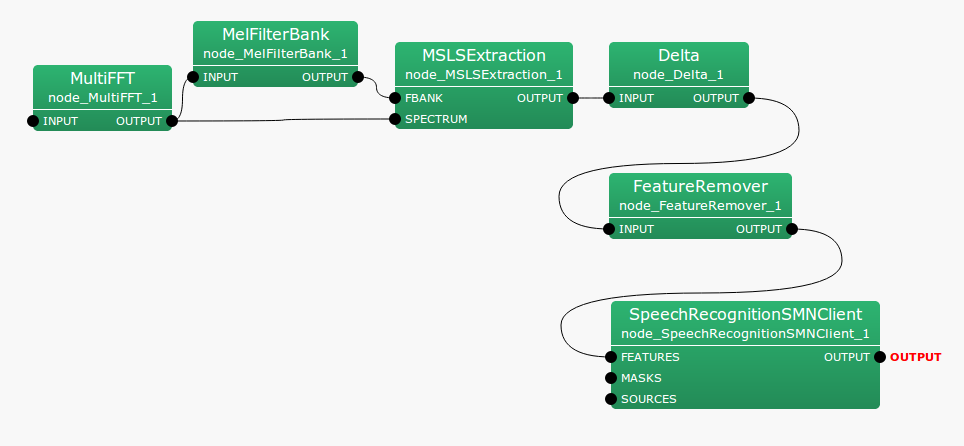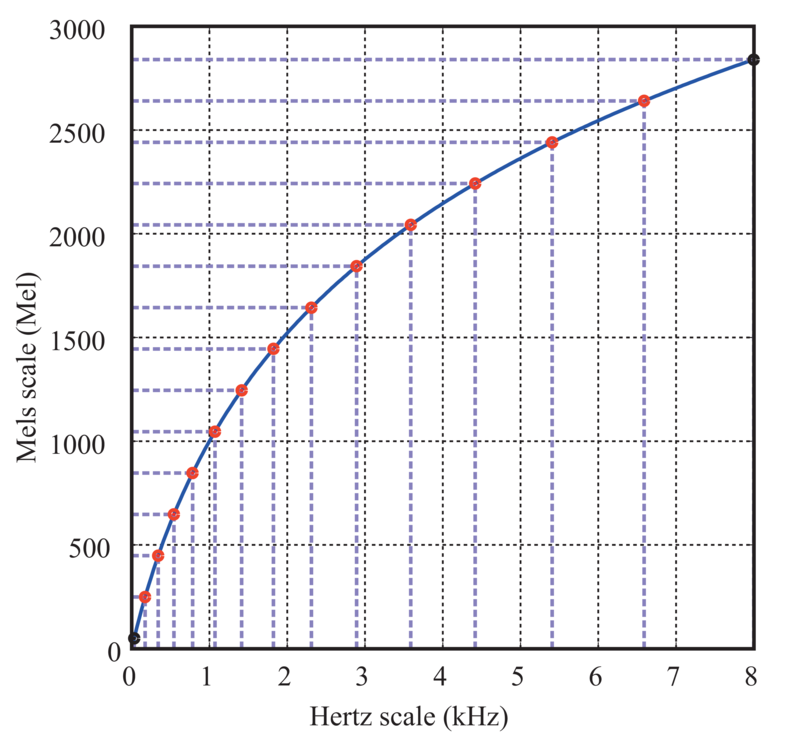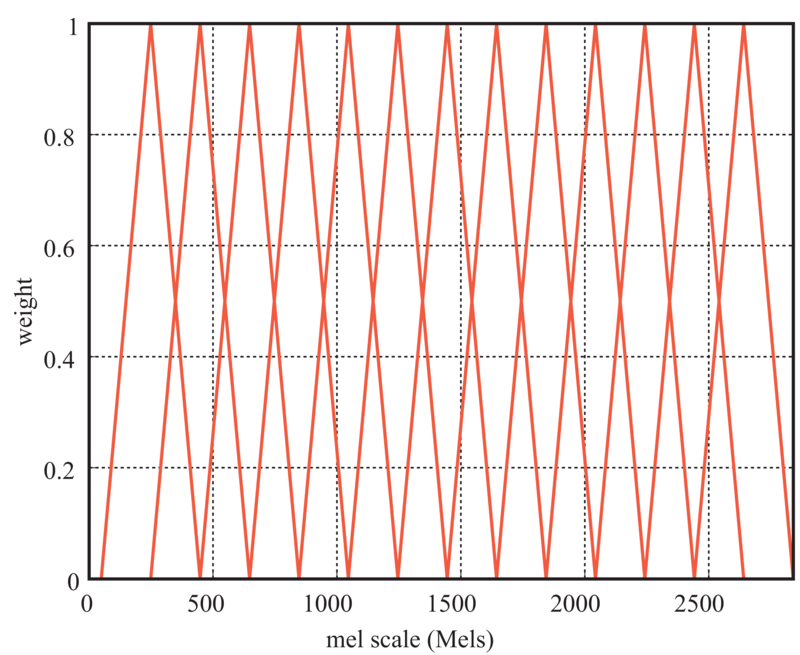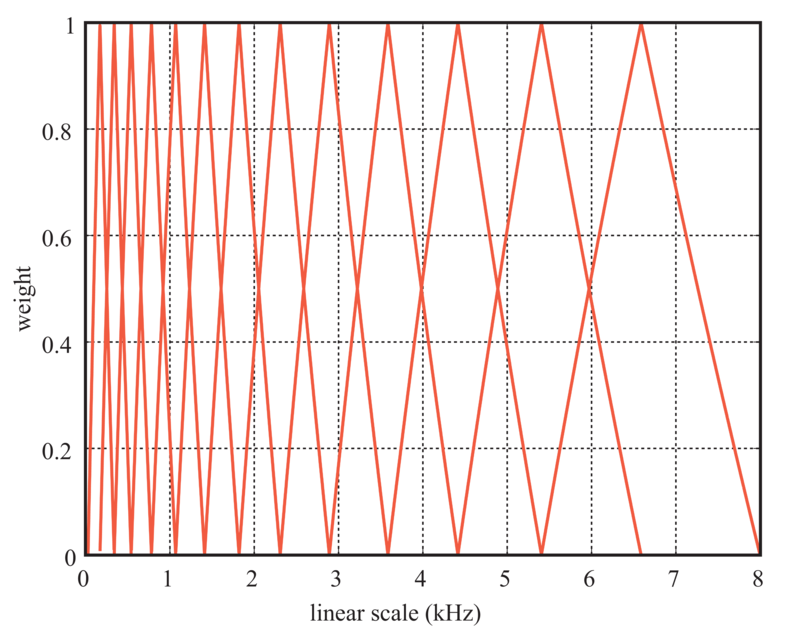## 6.4.3 MelFilterBank

### 6.4.3.1 Outline of the node

This node performs the mel-scale filter bank processing for input spectra and outputs the energy of each filter channel. Note that there are two types of input spectra, and output differs depending on inputs.

### 6.4.3.2 Necessary file

No files are required.

### 6.4.3.3 Usage

When to use

This node is used as preprocessing for acquiring acoustic features. It is used just after MultiFFT , PowerCalcForMap  or PreEmphasis . It is used before MFCCExtraction  or MSLSExtraction .

Typical connectionFigure 6.71: Connection example of MelFilterBank

### 6.4.3.4 Input-output and property of the node

Table 6.63: Parameter list of MelFilterBank
 Parameter name Type Default value Unit Description LENGTH 512 [pt] Analysis frame length SAMPLING_RATE 16000 [Hz] Sampling frequency CUT_OFF 8000 [Hz] Cut-off frequency of lowpass filter MIN_FREQUENCY 63 [Hz] Lower cut-off frequency of filter bank MAX_FREQUENCY 8000 [Hz] Upper limit frequency of filter bank FBANK_COUNT 13 Filter bank numbers

Input

INPUT

: Map<int, ObjectRef> type. A pair of the sound source ID and power spectrum as Vector<float> type or complex spectrum Vector<complex<float> > type data. Note that when the power spectrum is selected, output energy doubles, different from the case that the complex spectrum is selected.

Output

OUTPUT

: Map<int, ObjectRef> type. A pair of the sound source ID and the vector consisting of output energy of the filter bank as Vector<float> type data. The dimension number of output vectors is twice as large as FBANK_COUNT. Output energy of the filter bank is in the range from 0 to FBANK_COUNT-1 and 0 is in the range from FBANK_COUNT to 2 *FBANK_COUNT-1. The part that 0 is in is a placeholder for dynamic features. When dynamic features are not needed, it is necessary to delete with FeatureRemover .

Parameter

LENGTH

: int  type. Analysis frame length. It is equal to the number of frequency bins of the input spectrum. Its range is positive integers.

SAMPLING_RATE

: int  type. Sampling frequency. Its range is positive integers.

CUT_OFF

: Cut-off frequency of the anti-aliasing filter in a discrete Fourier transform. It is below 1/2 of SAMPLING_RATE.

MIN_FREQUENCY

: int  type. Lower cut-off frequency of the filter bank. Its range is positive integers and less than CUT_OFF.

MAX_FREQUENCY

: int  type. Upper limit frequency of the filter bank. Its range is positive integers and less than CUT_OFF.

FBANK_COUNT

: int  type. The number of filter banks. Its range is positive integers.

### 6.4.3.5 Details of the node

This node performs the mel-scale filter bank processing and outputs energy of each channel. Center frequency of each bank is positioned on mel-scale $^{(1)}$ at regular intervals. Center frequency for each channel is determined by performing FBANK_COUNT division from the minimum frequency bin $\hbox{SAMPLING\_ RATE}/\hbox{LENGTH}$ to $\hbox{SAMPLING\_ RATE} \hbox{CUT\_ OFF} / \hbox{LENGTH}$. Transformation of the linear scale and mel scale is expressed as follows.

 $\displaystyle m$ $\displaystyle =$ $\displaystyle 1127.01048 \log ( 1.0 + \frac{\lambda }{700.0} )$ (112)

However, expression on the linear scale is \lambda (Hz) and that on the mel scale is $m$. Figure 6.72 shows an example of the transformation by 8000 Hz. The red points indicate the center frequency of each bank when SAMPLING_RATE is 16000Hz, CUT_OFF is 8000Hz and FBANK_COUNT is 13. The figure shows that the center frequency of each bank is at regular intervals on the mel scale.Figure 6.72: Correspondence between linear scale and a mel scale

Figure 6.73 shows the window functions of the filter banks on the mel scale. It is a triangle window that becomes 1.0 on the center frequency parts and 0.0 on the center frequency parts of adjacent channels. Center frequency for each channel is at regular intervals on the mel scale and in symmetric shape. These window functions are represented as shown in Figure 6.74 on the linear scale. A wide band is covered in high frequency channels.Figure 6.73: Window function on mel scaleFigure 6.74: Window function on linear scale

The input power spectrum expressed on the linear scale is weighted with the window functions shown in Figure 6.74 and energy is obtained for each channel and output.

### 6.4.3.6 References:

(1) Stanley Smith Stevens, John Volkman, Edwin Newman: “A Scale for the Measurement of the Psychological Magnitude Pitch”, Journal of the Acoustical Society of America 8(3), pp.185–190, 1937.